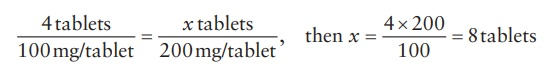# Ratio and proportion

| Home | | Pharmaceutical Drugs and Dosage | | Pharmaceutical Industrial Management |

## Chapter: Pharmaceutical Drugs and Dosage: Pharmacy math and statistics

Ratio represents a quantitative relationship between two quantities.

Ratio and proportion

Ratio represents a quantitative relationship between two quantities. It can be expressed as a fraction (e.g., ½, ¼, and so on) or as a ratio (e.g., 1:2, 1:4, and so on).

A proportion represents the equality of two ratios. Thus,

1/2=2/4

The equality of two ratios can be checked by cross multiplying the numerator of the first with the denominator of the second. For example,

1/2 = 2/4 but 1/2 ≠ 2/6

because

1× 4 = 2 × 2 but 1× 6 2 × 2

Proportions are commonly utilized to find an unknown quantity or variable when three other related quantities or variables are known. For example,

If x/4 = 2/9 , then x = (2 × 4)/9 = 8/9

In these calculations, caution must be exercised to ensure that the numera-tors and denominators have the same units on both sides of the proportion. For example, if the pharmacist wishes to substitute 100-mg strength tablets with 200-mg strength tablets for a patient who was prescribed four tablets of 100-mg strength, then the number of tablets of 200-mg strength can be calculated as:A good practice in carrying out these calculations is to always label the units in the proportions.